# R for Data Science Interview Questions Data Science

Prepare better with the best interview questions and answers, and walk away with top interview tips. These interview questions and answers will boost your core interview skills and help you perform better. Be smarter with every interview.

• 4.6 Rating
• 39 Question(s)

## Beginner

Matplot  plots the columns of a matrix individually as a function of x. syntax of matplot is matplot(x, cbind(y1,y2)) where x, y1,y2 are vectors or columns of a matrix or dataframe. Example as shown below where X is the vector from 1 to 8 and Y1 and Y2 are functions of X.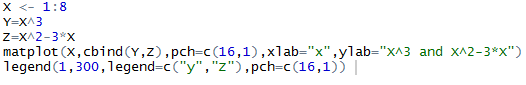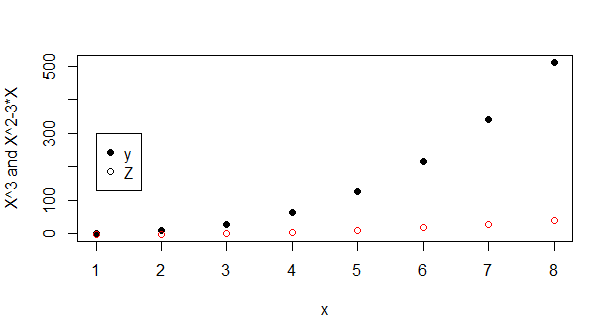apply(). apply( ) function is used on arrays. lapply( ) function can be used on objects like dataframes, lists or vectors and the output would be a list again whereas sapply() function is used to simplify the results of lapply. For example, list of lapply( ) is simplified as vector in sapply( ).  mapply( ) stands for ‘multivariate’ apply which is used on multiple lists or multiple vector argument.

Min(). You can find the minimum and the maximum of a vector using the min() or the max() function. A function called range() is also available which returns the minimum and maximum in a two-element vector. If we you to find where the minimum or maximum is located, i.e. the index instead of the actual value, then you can use which.min() and which.max() functions.

Legend function adds legend box to the plot which makes the graph more simpler and interpretable. legend(x, y = NULL, legend, fill = NULL, col = par("col"), border= "black", lty, lwd, pch) where x and y are the coordinates which is used to position the legend. legend, a character vector of legend names. fill, fill legend box with specified colors. col, the color of points or lines appearing in the legend. border, color for the legend box border. lty and lwd, the line types and widths for the line in the legend box. pch, plotting symbols in the legend.

Stacking vectors concatenates multiple vectors into a single vector along with a factor indicating where each observation originated. Unstacking reverses this operation.

Example: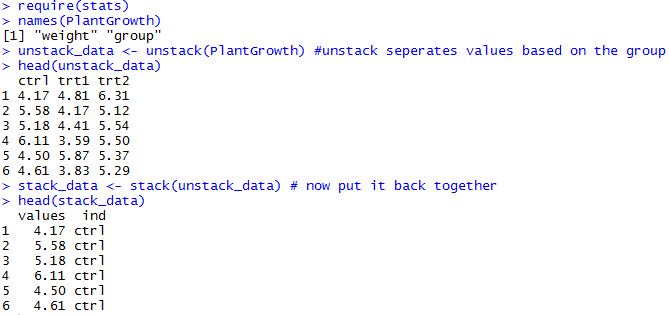unlist() is the function used to convert list into vector for arithmetic manipulation on the elements.

The class() is used to define/identify what "type" an object is from the point of view of object-oriented programming in R. Generally class function is used for identifying the dataframe object. typeof() gives the "type" of object from R's point of view, while mode() gives the "type" of object from the point of view of Becker, Chambers & Wilks. Data.frame() is used to create dataframe objects.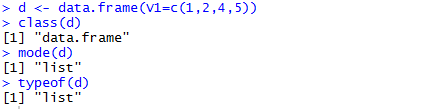df<- Data.frame(x, StringsAsFactors = FALSE). stringsAsFactorsFALSE should be passed in the dataframe argument in order to suppress the conversion. By default, no argument in the function is taken as StringsAsFactors = TRUE.

True. For example, ‘b’ is the data to be split by the group defined by ‘a’. The output ‘s’ shows two different groups.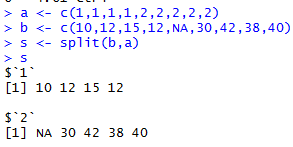True. Using vector function improves memory usage and increases speed than c() function. Example as shown below.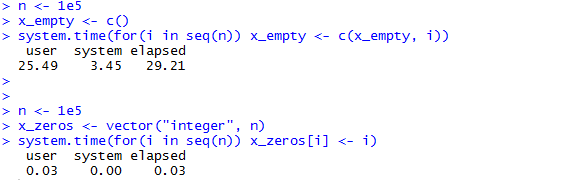sub() function replaces only the first occurrence of a substring whereas gsub() is the global replace function, which replaces all instances of the substring.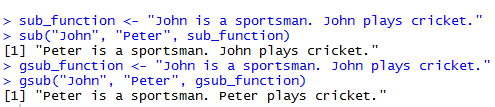initial <- read.table(“datatable.txt”, nrows = 20, header = FALSE,  sep="/", strip.white = TRUE, na.strings = "EMPTY"). The nrows function allows you to specify the number of rows to be imported from the file. strip.white argument allows you to indicate whether you want the leading and trailing white spaces from unquoted character fields stripped(numeric fields are always stripped) and only takes on a logical value. The na.strings indicates which strings should be interpreted as NA values. colClasses allows you to specify a vector of classes for all columns of your data set.

Yes, there is a difference. paste() is like concatenation using separation factor, whereas, paste0() is like append function using separation factor. For example,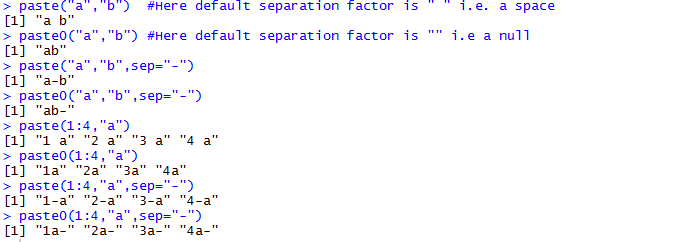False. A factor values can be deleted with their levels. The simplest solution is often just to wrap it in factor () again. For example, let’s consider a factor with four levels and delete level “c” and factor it again. You will see level “c” is dropped.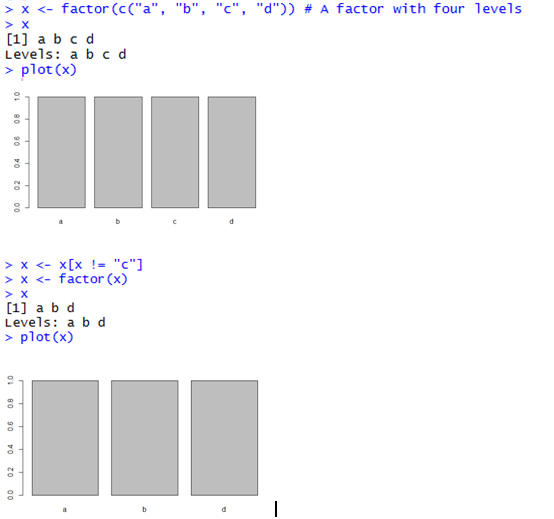fread is faster than read.csv. read.csv(filename) creates dataframe and it is slow mainly because it first reads everything into memory as if it were character and then attempts to coerce that to integer or numeric as a second step and also reads the file into a buffer via a connection whereas fread creates data table and maps the file as character into memory and then iterates through the file using pointers.

plot(x, y, main, xlab, ylab, xlim, ylim, axes)  where x and y are the data set whose values are the horizontal coordinates and vertical coordinates respectively. main is the tile of the graph. xlab and ylab are the labels in the horizontal and vertical respectively. xlim and ylim are the limits of the values of x and y used for plotting respectively. axes indicates whether both axes should be drawn on the plot.

strsplit() is used to split a string to calculate the used keywords in the list. The str_detect() function helps to check whether a substring exists in a string. It returns TRUE/FALSE against each value. You can use strrep function to repeat the character N times.

You can use the rep() function to repeat the complete vector. Seq() function generates a sequence of numbers. Any() takes the set of vectors and returns a set of logical vectors, in which at least one of the values true. All() takes the set of vectors and returns a set of logical vectors, in which all of the values true.

It can be done using the match() function which returns the first appearance of a particular element. The other way is %in% which returns a Boolean value either true or false. Is.element() function also returns a Boolean value either true or false based on whether it is present in a vector or not.

False. rnorm(n, mean = , sd = ) is used to generate n normal random numbers with arguments mean and sd whereas runif(n, min = , max = ) is used to generate n uniform random numbers lie in the interval (min, max)

grep returns a vector of the indices of the elements of x that yielded a match whereas grepl returns a logical vector based on the matching elements. For example,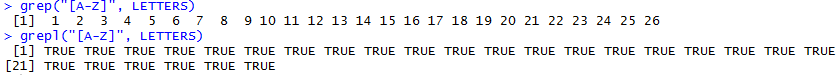[] returns multiple elements of data. The index within the square brackets can be a numeric vector, a logical vector, or a character vector. [[]] extracts elements within the list and it offers the flexibility of referring to the elements by position rather than by name. It can be used for data frames and lists. Let’s see with examples in different scenarios. The dollar sign operator selects a single element of data. When you use this operator with a data frame, the result is always a vector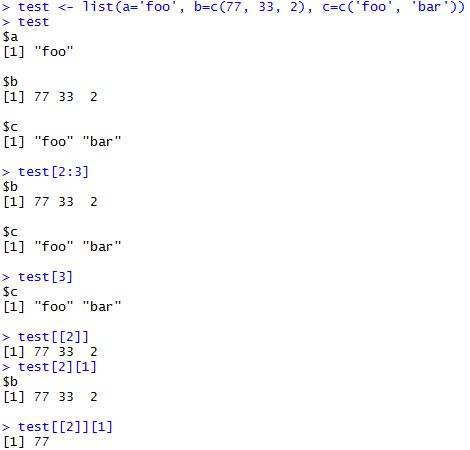merge() function is used to merge two data frames. The data frames must have same column names on which the merging happens. You can join two data frames by one or more common key variables (i.e., an inner join). cbind() function is used to combine vector, matrix or dataframe by columns. rbind() function is used to combine vector, matrix or dataframe by rows.

X[is.na(X)] is used to select the values that are NA whereas X[!is.na(X)] is used to remove the NA from the vector.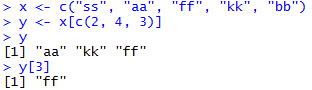head(subset(airquality, Ozone > 28 & Temp > 70, select=c(Ozone, Temp)), 4)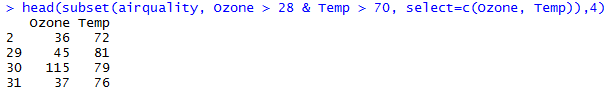subset(airquality, !is.na(Ozone))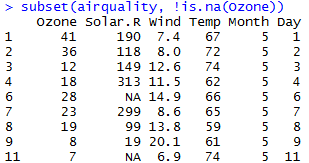head(subset(airquality, Month==5 & Day > 30, select= -Wind))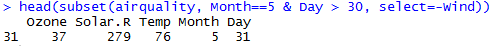Yes, Cumulative statistics in R are applied sequentially to a series of values. For example, to track the interest received on an investment, cumulative statistics are used. Cumulative commands produce an accurate result when applies to a vector of character data. However, if applied on character data, they give error populated as a list of NA items. If numeric vector contains NA, the cumulative command will work till first NA and thereafter give all result as NA. Cumsum(x): The cumulative sum of a vector. Cummax(x): The cumulative maximum value. Cumin(x): The cumulative minimum value. Cumprod(x): The cumulative product.

Rowmeans() command gives the mean of values in the row while rowsums() command gives the sum of values in the row. Similarly, colmeans() and colsums() are applied to column data.

Yes. rbind.fill from the package plyr,  bind_rows from the package dplyr and smartbind from gtools does the job.

To convert an object into a table, you can use the as.table() command if it is already a matrix; however, if it is a data frame, you need to first convert it to a matrix and then convert into a table.

In R, Cross-tabulation means representing raw data into a tabular format For cross tabulation, you can use the xtabs() command as follows: xtabs(freq.data~categories.list, data)

Set.seed(500);train<- createDataPartition(p=0.8, list=FALSE, iris\$Species).  seed is an integer vector, containing the random number generator (RNG) state for random number generation in R. createDataPartition is used to split the data. createDataPartition(y, times = 1, p = 0.5, list = TRUE, groups = min(5, length(y))) where y is the vector of outcomes, Times, the number of partitions to create, list, logical - should the results be in a list (TRUE) or a matrix with the number of rows equal to floor(p * length(y)) and times columns and groups, for numeric y, the number of breaks in the quantiles.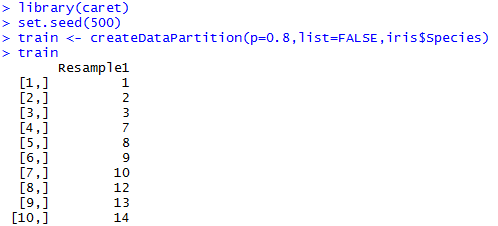createDataPartition(p=0.8,list=FALSE, times=5, iris\$Species) where time specifies the different number of samples to be generated.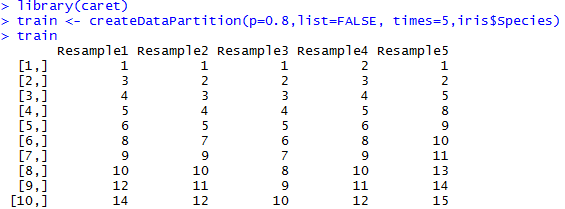createFolds(iris\$Species,k=5). createFolds is used to create folds/partition given the features matrix where iris\$Species represents the feature to be partitioned and k represents the number of folds.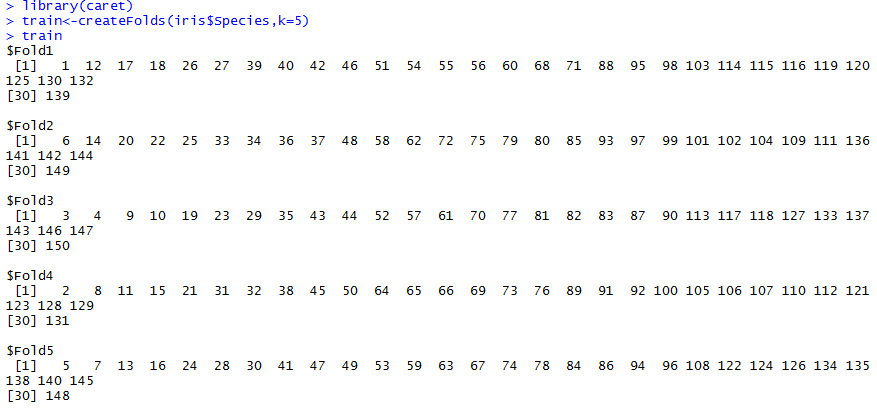mapply(rep, 1:10, 5). mapply applies a function to the first elements of each argument, the second elements, the third elements, and so on.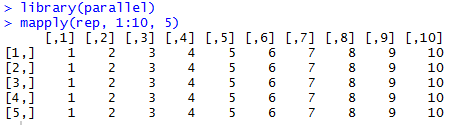mapply(paste,1:3, 6:8, sep=letters[24:26])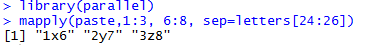## Description

Prepare better with the best interview questions and answers, and walk away with top interview tips. These interview questions and answers will boost your core interview skills and help you perform better. Be smarter with every interview.